# Broadcasting in Python

In this post, we will discuss ‘Broadcasting’ using NumPy. It is also used while implementing neural networks as these operations are memory and computationally efficient.

So let’s understand what Broadcasting means followed by a few examples!

Broadcasting describes the way numpy treats arrays with different shapes for arithmetic operations. The smaller array is broadcasted across the larger array so that they have compatible shapes. It provides a way to vectorize array operations thus leading to efficient implementations.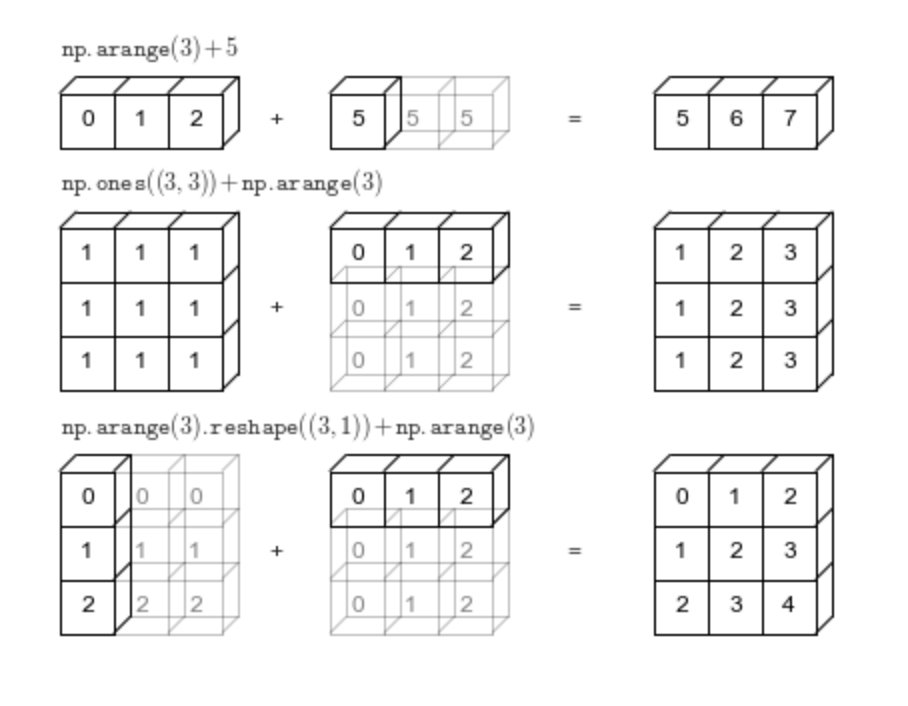Broadcasting Examples- Image Source

The light bordered boxes represent the broadcasted values, this extra memory is not actually allocated during the operation, but can be useful conceptually to imagine that it is.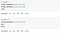Normal arithmetic operation and broadcasting comparison

In the above example for both cases output is same while the second case uses the concept of broadcasting and is more efficient. NumPy is elegant enough to use the original scalar value without actually making copies making broadcasting operations more efficient.

# More Broadcasting examples

• Example 1- Arithmetic operation between two arrays where the first array has shape (m,n) and the second array with shape (m,1).

Output: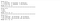Final Output shape is (2,3) corresponding to ‘a’
• Example 2- Arithmetic operation between two arrays where the first array has shape (m,n) and the second array with shape (1,n).

Output: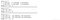Final Output shape is (2,3) corresponding to ‘a’
• Example 3- Arithmetic operation between an array and scalar value where the array has shape (1,n)

Output: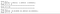Final Output shape is (1,4) corresponding to ‘a’
• Example 4- Arithmetic operation between an array and scalar value where the array has shape (m,1).

Output: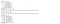Final Output shape is (4,1) corresponding to ‘a’

In the examples above I have used ‘+’ arithmetic operation to demonstrate Broadcasting, it can be replicated for other arithmetic operators too in a similar fashion.

So that's it! You have made it to the end of this quick demo of Broadcasting in Python.

References:

Data Scientist. LinkedIn — https://www.linkedin.com/in/pooja-mahajan-69b38a98/.